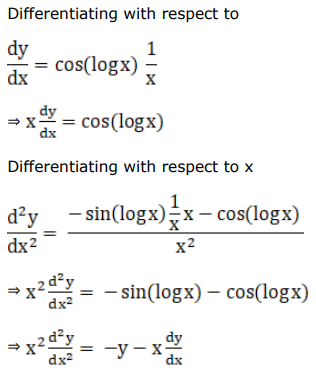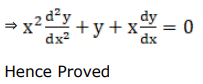# Solve this followingQuestion:

If $y=\sin (\log x)$, prove that $x^{2} \frac{d^{2} y}{d x^{2}}+x \frac{d y}{d x}+y=0$

Solution: